## Stoichiometry Worksheet PDF Details

When it comes to learning chemistry, one of the most important things students must learn is stoichiometry. A great way to practice this skill is by using stoichiometry worksheets. These sheets can help students master the calculations necessary for solving problems related to chemical reactions. In addition, worksheets can also help students become better problem-solvers. There are many different types of stoichiometry worksheets available online, so finding the right one for your needs should be easy. However, before choosing a worksheet, it is important to understand what type you need. This article will discuss the different types of stoichiometry worksheets and how to choose the right one for your needs.

You'll discover info about the type of form you want to complete in the table. It will tell you how much time you'll need to fill out stoichiometry worksheet, what parts you will have to fill in, and so on.

Form NameStoichiometry Worksheet
Form Length4 pages
Fillable?No
Fillable fields0
Avg. time to fill out1 min
Other namesFe203, beginning stoichiometry worksheet, stoichiometry worksheet, stoichiometry worksheet na2sio3

## Form Preview ExampleStoichiometry Worksheet and Key

2 KClO3 è 2 KCl + 3 O2

1. How many moles of O2 will be formed from 1.65 moles of KClO3?

 1.65 mol KClO3 mol O2 mol O2 = mol KClO3

2. How many moles of KClO3 are needed to make 3.50 moles of KCl?

3.50mol KCl

= mol KClO3

3. How many moles of KCl will be formed from 2.73 moles of KClO3?

=

4 Fe + 3 O2 è 2 Fe2O3

4. How many moles of Fe2O3 are produced when 0.275 moles of Fe is reacted?

0.275 mol Fe

= mol Fe2O3

5. How many moles of Fe2O3 are produced when 31.0 moles of O2 is reacted?

=

6. How many moles of O2 are needed to react with 8.9 moles of Fe?

=

2H2O è 2 H2 + O2

7.How many moles of O2 are produced when 1.26 moles of H2O is reacted?

8.How many moles of H2O are needed to produce 55.7 moles of H2?

9.If enough H2O is reacted to produce 3.40 moles of H2, then how may moles of O2 must have been made? (a bit challenging, but just think about it and you can probably figure it out)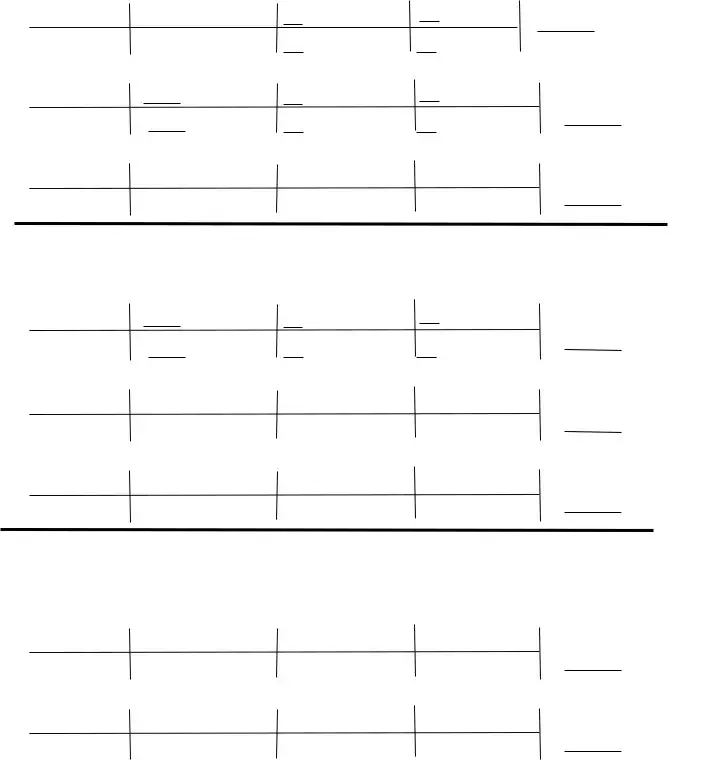2 KClO3 è 2 KCl + 3 O2

 10. How many grams of O2 will be formed from 3.76 grams of KClO3? 3.76g KClO3 1 mol KClO3 mol O2 g O2 = g O2 122.55 g KClO3 mol KClO3 mol O2

11. How many grams of KClO3 are needed to make 30.0 grams of KCl?

 30.0 g KCl mol KCl mol KClO3 g KClO3 = g KCl mol KCl mol KClO3

12.How many grams of KCl will be formed from 2.73 g of KClO3?

2.73g KClO3

=

4 Fe + 3 O2 è 2 Fe2O3

13. How many grams of Fe2O3 are produced when 42.7 grams of Fe is reacted?

 42.7 g Fe mol Fe mol Fe2O3 g Fe2O3 g Fe mol Fe = mol Fe2O3

14. How many grams of Fe2O3 are produced when 17.0 grams of O2 is reacted?

 17.0 g O2 =

15. How many grams of O2 are needed to react with 125 grams of Fe?

=

Some cars can use butane (C4H10) as fuel:

 2 C4H10 + 13 O2 è 8 CO2 + 10 H2O 16. How many grams of CO2 are produced from the combustion of 100. grams of butane? 100. g C4H10 = 17. How many grams of O2 are needed to react with of 100. grams of butane? 100. g C4H10 = 18 How many grams of H2O are produced when 5.38g of O2 is reacted?

g KClO3

g KCl

g Fe2O3

g Fe2O3

g CO2

g O2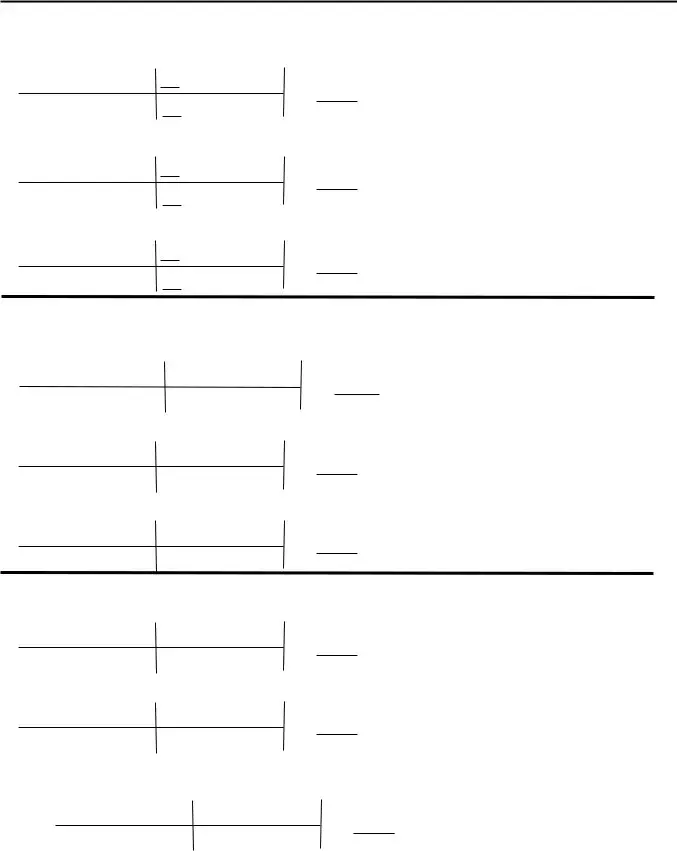2 KCl + 3 O2
2 KClO3

KEY

è

1. How many moles of O2 will be formed from 1.65 moles of KClO3?

 1.65 mol KClO3 3 mol O2 2.48 mol O2 = 2 mol KClO3

2. How many moles of KClO3 are needed to make 3.50 moles of KCl?

 3.50 mol KCl 2 mol KClO3 mol KClO3 = 3.50 2 mol KCl

3. How many moles of KCl will be formed from 2.73 moles of KClO3?

 2.73 moles KClO3 2 mol KCl 2.73 mol KCl = 2 mol KClO3 4 Fe + 3 O2 è 2 Fe2O3

4. How many moles of Fe2O3 are produced when 0.275 moles of Fe are reacted?

 0.275 mol Fe 2 mol Fe2O3 = 0.138 mol Fe2O3 4 mol Fe

5. How many moles of Fe2O3 are produced when 31.0 moles of O2 are reacted?

 31.0 mol O2 2 mol Fe2O3 = 20.7 mol Fe2O3 3 mol O2

6. How many moles of O2 are needed to react with 8.9 moles of Fe?

 8.9 mol Fe 3 mol O2 = 6.7 mol O2 4 mol Fe

2H2O è 2 H2 + O2

7.How many moles of O2 are produced when 1.26 moles of H2O is reacted?

1.26mol H2O 1 mol O2= .630 mol O2 2 mol H2O

8.How many moles of H2O are needed to produce 55.7 moles of H2?

55.7mol H2 2 mol H2O = 55.7 mol H2O 2 mol H2

9.If enough H2O is reacted to produce 3.40 moles of H2, then how may moles of O2 must have been made? (a bit challenging, but just think about it and you can probably figure it out)

 3.40 mol H2 1 mol O2 1.70 mol O2 = 2 mol H22 KClO3 è 2 KCl + 3 O2

 10. How many grams of O2 will be formed from 3.76 grams of KClO3? 3.76g KClO3 1 mol KClO3 3 mol O2 32.00 g O2 = 1.47 g O2 122.55 g KClO3 2 mol KClO3 1 mol O2 11. How many grams of KClO3 are needed to make 30.0 grams of KCl? 30.0 g KCl 1 mol KCl 2 mol KClO3 122.55 g KClO3 = 49.3 g KClO3 74.55 g KCl 2 mol KCl 1 mol KClO3

12. How many grams of KCl will be formed from 2.73 g of KClO3?

 2.73 g KClO3 1 mol KCl O3 2 mol KCl 74.55 g = 1.66 g KCl 122.55 g KClO3 2 mol KCl O3 1 mol KCl

4 Fe + 3 O2 è 2 Fe2O3

13. How many grams of Fe2O3 are produced when 42.7 grams of Fe is reacted?

 42.7 g Fe 1 mole Fe 2 mol Fe2O3 159.70 g Fe2O3 = 61.0 g Fe2O3 55.85 g Fe 4 mol Fe 1 mol Fe2O3 14. How many grams of Fe2O3 are produced when 17.0 grams of O2 is reacted? = 56.6 g Fe2O3 17.0 g O2 1 mol O2 2 mol Fe2O3 159.70 g Fe2O3 32.00 g O2 3 mol O2 1 mol Fe2O3

15. How many grams of O2 are needed to react with 125 grams of Fe?

 125 g Fe 1 mol Fe 3 mol O2 32.00 g O2 = 53.7 g O2 55.85 g Fe 4 mol Fe 1 mol O2 g Some cars can use butane (C4H10) as fuel: 2 C4H10 + 13 O2 è 8 CO2 + 10 H2O 16. How many grams of CO2 are produced from the combustion of 100. grams of butane? 100. g C4H10 1 mol C4H10 8 mol CO2 44.01 g CO2 = 303 g CO2 58.14 g C4H10 2 mol C4H10 1 mol CO2

17. How many grams of O2 are needed to react with of 100. grams of butane?

 100. g C4H10 1 mol C4H10 13 mol O2 32.00 g O2 = 358 g O2 58.14 g C4H10 2 mol C4H10 1 mol O2 18 How many grams of H2O are produced when 5.38g of O2 is reacted? 5.38g O2 1 mol O2 10 mol H2O 18.02 g H2O = 2.33 g H2O 32.00 g O2 13 mol O2 1 mol H2O

This PDF editor makes it simple to manage the C6HsBr form. It's possible to prepare the form without delay through using these simple steps.

Step 1: Locate the button "Get Form Here" and select it.

Step 2: You can now edit the C6HsBr. Our multifunctional toolbar helps you include, remove, change, and highlight content material or perhaps carry out other sorts of commands.

If you want to prepare the C6HsBr PDF, provide the content for each of the segments: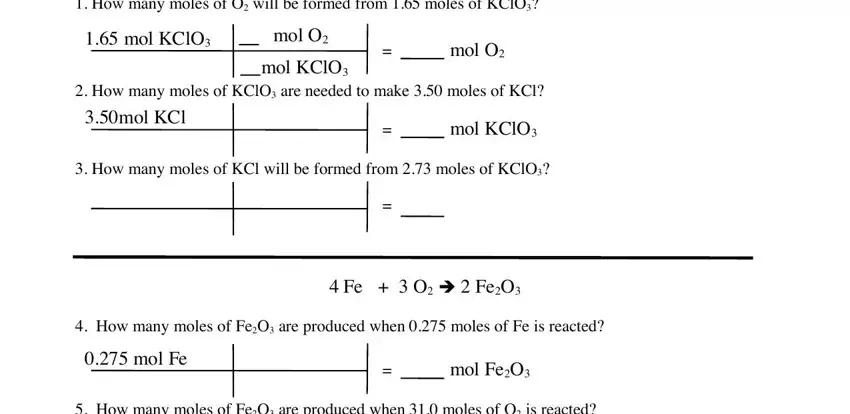Complete the How many moles of FeO are, How many moles of O are needed to, HO è H O, How many moles of O are produced, and How many moles of HO are needed fields with any content that may be asked by the platform.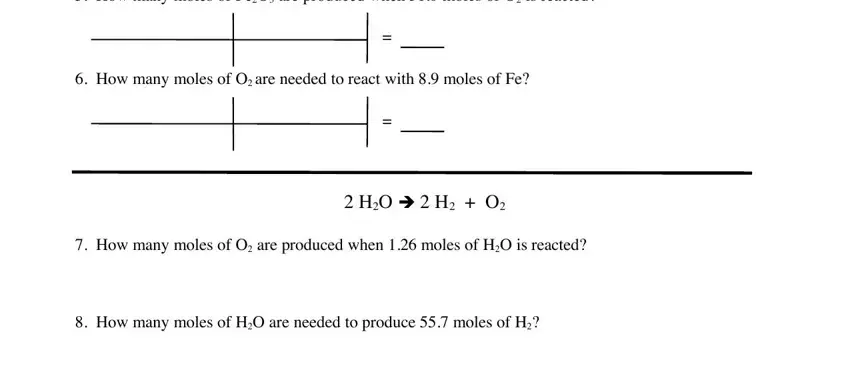The system will ask you for data to automatically fill up the section How many grams of O will be, g KClO, mol KClO g KClO, mol O mol KClO, g O mol O, g O, How many grams of KClO are needed, g KCl, mol KCl, mol KClO, g KClO, How many grams of KCl will be, g KCl, mol KCl, and mol KClO.You will have to define the rights and obligations of every party in box How many grams of FeO are, g O, How many grams of O are needed to, g FeO, Some cars can use butane CH as fuel, CH O è CO HO, How many grams of CO are produced, g CH, How many grams of O are needed to, g CH, and g CO.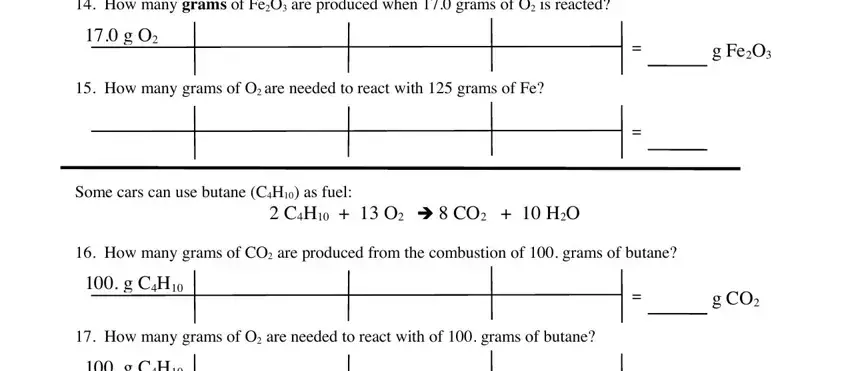Finalize by looking at the following areas and completing them as required: g CH, How many grams of HO are produced, and g O.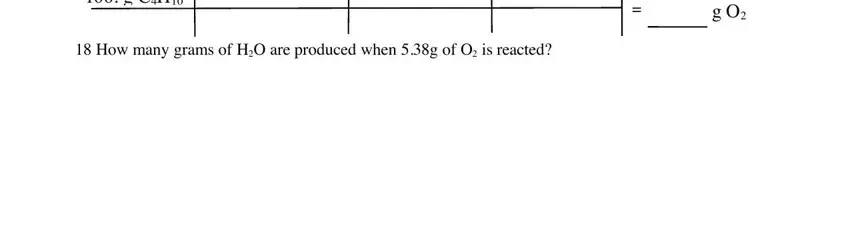Step 3: Select the Done button to be sure that your completed document may be exported to any type of electronic device you use or delivered to an email you specify.

Step 4: Make copies of the file - it will help you stay away from forthcoming issues. And don't get worried - we cannot distribute or look at your information.

## Watch Stoichiometry Worksheet Video Instruction